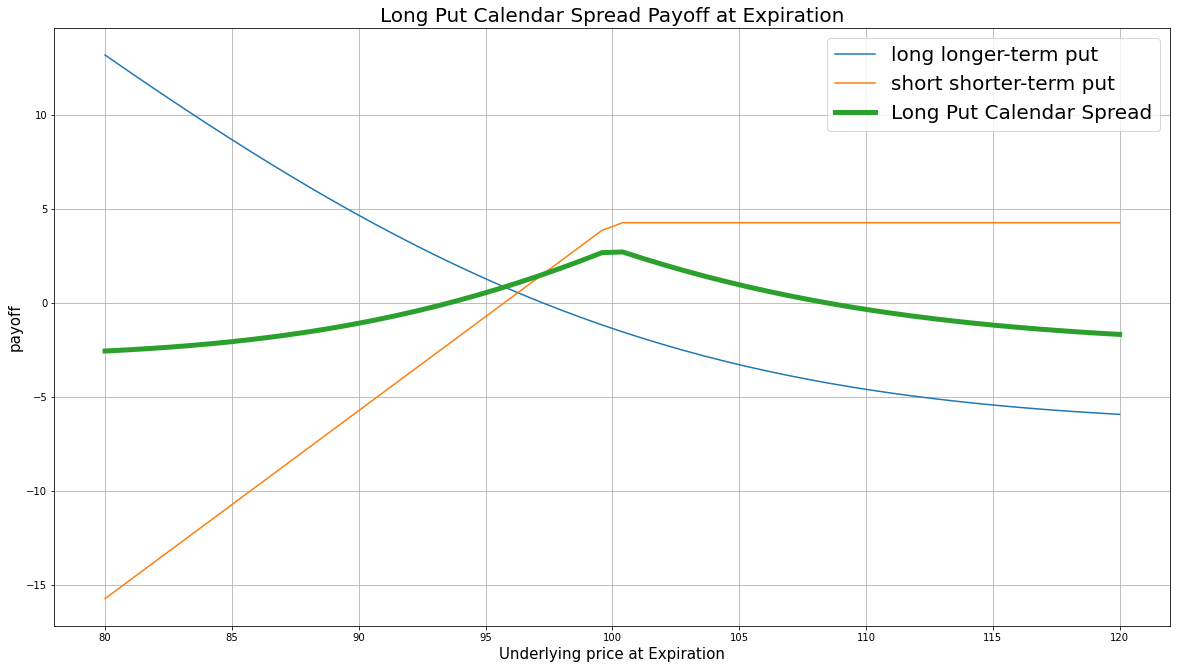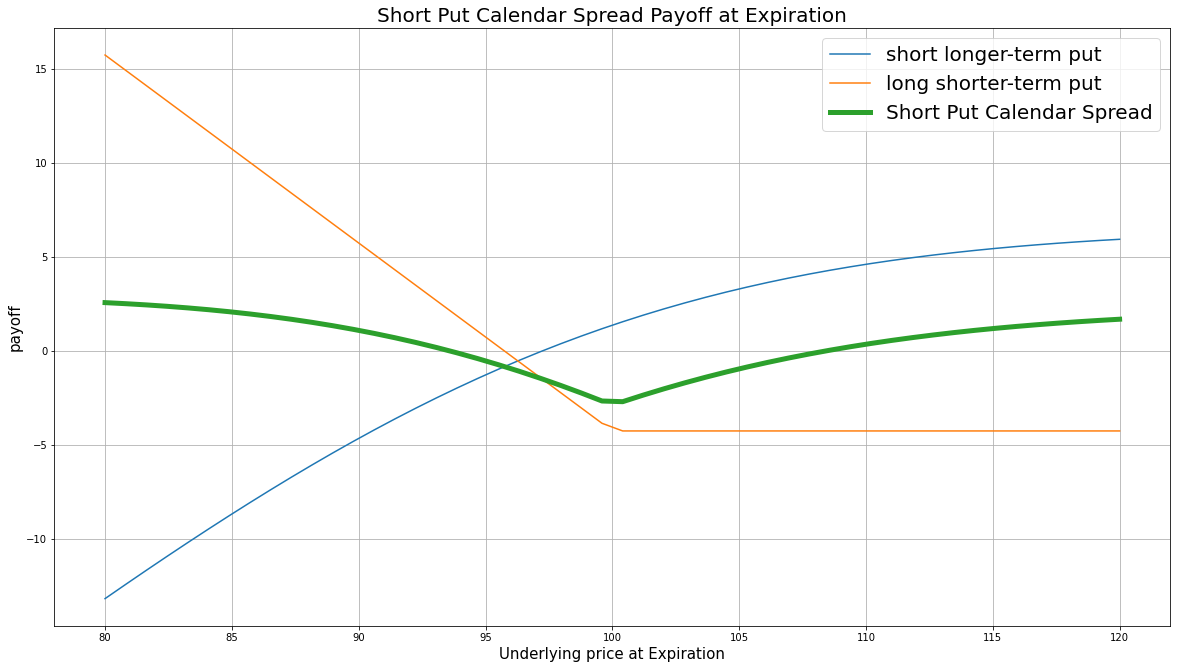# Option Strategies

### Introduction

Put calendar spread, also known as put horizontal spread, is a combination of a longer-term (far-leg/front-month) put and a shorter-term (near-leg/back-month) put, where both puts have the same underlying stock and the same strike price. The put calendar spread strategy can be long or short.

The long put calendar spread consists of buying a longer-term put and selling a shorter-term put. This strategy profits from a decrease in price movement. The strategy also profits from the time decay value because the theta $\theta$ (the Option price decay by 1 day closer to maturity) of the shorter-term put is larger than the longer-term put.

The short put calendar spread consists of selling a longer-term put and buying a shorter-term put. This strategy profits from an increase in price movement.

### Implementation

1. In the Initialize method, set the start date, end date, cash, and Option universe.
2. private Symbol _symbol;

public override void Initialize()
{
SetStartDate(2017, 2, 1);
SetEndDate(2017, 2, 19);
SetCash(500000);

_symbol = option.Symbol;
option.SetFilter(universe => universe.IncludeWeeklys()
.Strikes(-1, 1)
.Expiration(TimeSpan.FromDays(0), TimeSpan.FromDays(62)));
}
def Initialize(self) -> None:
self.SetStartDate(2017, 2, 1)
self.SetEndDate(2017, 2, 19)
self.SetCash(500000)

self.symbol = option.Symbol
option.SetFilter(self.UniverseFunc)

def UniverseFunc(self, universe: OptionFilterUniverse) -> OptionFilterUniverse:
return universe.Strikes(-1, 1).Expiration(timedelta(0), timedelta(62))
3. In the OnData method, select the strike price and expiration dates of the contracts in the strategy legs.
4. public override void OnData(Slice slice)
{
if (Portfolio.Invested) return;

// Get the OptionChain
var chain = slice.OptionChains.get(_symbol, null);
if (chain == null || chain.Count() == 0) return;

// Get the ATM strike price
var atmStrike = chain.OrderBy(x => Math.Abs(x.Strike - chain.Underlying.Price)).First().Strike;

// Select the ATM put contracts
var puts = chain.Where(x => x.Strike == atmStrike && x.Right == OptionRight.Put);
if (puts.Count() == 0) return;

// Select the near and far expiration dates
var expiries = puts.Select(x => x.Expiry).OrderBy(x => x);
var nearExpiry = expiries.First();
var farExpiry = expiries.Last();
def OnData(self, slice: Slice) -> None:
if self.Portfolio.Invested: return

# Get the OptionChain
chain = slice.OptionChains.get(self.symbol, None)
if not chain: return

# Get the ATM strike price
atm_strike = sorted(chain, key=lambda x: abs(x.Strike - chain.Underlying.Price)).Strike

# Select the ATM put contracts
puts = [i for i in chain if i.Strike == atm_strike and i.Right == OptionRight.Put]
if len(puts) == 0: return

# Select the near and far expiration dates
expiries = sorted([x.Expiry for x in puts], key = lambda x: x)
near_expiry = expiries
far_expiry = expiries[-1]
5. In the OnData method, call the OptionStrategies.PutCalendarSpread method and then submit the order.
6. var optionStrategy = OptionStrategies.PutCalendarSpread(_symbol, atmStrike, nearExpiry, farExpiry);
Sell(optionStrategy, 1);   // if short put calendar spread
option_strategy = OptionStrategies.PutCalendarSpread(self.symbol, atm_strike, near_expiry, far_expiry)
self.Sell(option_strategy, 1)   # if short put calendar spread

Option strategies synchronously execute by default. To asynchronously execute Option strategies, set the asynchronous argument to Falsefalse. You can also provide a tag and order properties to the Buy and Sell methods.

Buy(optionStrategy, quantity, asynchronous, tag, orderProperties);
Sell(optionStrategy, quantity, asynchronous, tag, orderProperties);

self.Buy(option_strategy, quantity, asynchronous, tag, order_properties)
self.Sell(option_strategy, quantity, asynchronous, tag, order_properties)


### Strategy Payoff

The put calendar spread can be long or short.

The long put calendar spread is a limited-reward-limited-risk strategy. The payoff is taken at the shorter-term expiration. The payoff is

$$\begin{array}{rcll} P^{\textrm{short-term}}_T & = & (K - S_T)^{+}\\ P_T & = & (P^{\textrm{long-term}}_T - P^{\textrm{short-term}}_T + P^{\textrm{short-term}}_0 - P^{\textrm{long-term}}_0)\times m - fee \end{array}$$ $$\begin{array}{rcll} \textrm{where} & P^{\textrm{short-term}}_T & = & \textrm{Shorter term put value at time T}\\ & P^{\textrm{long-term}}_T & = & \textrm{Longer term put value at time T}\\ & S_T & = & \textrm{Underlying asset price at time T}\\ & K & = & \textrm{Strike price}\\ & P_T & = & \textrm{Payout total at time T}\\ & P^{\textrm{short-term}}_0 & = & \textrm{Shorter term put value at position opening (credit received)}\\ & P^{\textrm{long-term}}_0 & = & \textrm{Longer term put value at position opening (debit paid)}\\ & m & = & \textrm{Contract multiplier}\\ & T & = & \textrm{Time of shorter term put expiration} \end{array}$$

The following chart shows the payoff at expiration:The maximum profit is undetermined because it depends on the underlying volatility.  It occurs when $S_T = S_0$ and the spread of the puts are at their maximum.

The maximum loss is the net debit paid, $P^{\textrm{short-term}}_0 - P^{\textrm{long-term}}_0$. It occurs when the underlying price moves very deep ITM or OTM so the values of both puts are close to zero.

If the Option is American Option, there is risk of early assignment on the sold contract. Naked long puts pose risk of losing all the debit paid if you don't close the position with short put together and the price drops below its strike.

The short put calendar spread is a limited-reward-limited-risk strategy. The payoff is taken at the shorter-term expiration. The payoff is

$$\begin{array}{rcll} P^{\textrm{short-term}}_T & = & (K - S_T)^{+}\\ P_T & = & (P^{\textrm{short-term}}_T - P^{\textrm{long-term}}_T + P^{\textrm{long-term}}_0 - P^{\textrm{short-term}}_0)\times m - fee \end{array}$$ $$\begin{array}{rcll} \textrm{where} & P^{\textrm{short-term}}_T & = & \textrm{Shorter term put value at time T}\\ & P^{\textrm{long-term}}_T & = & \textrm{Longer term put value at time T}\\ & S_T & = & \textrm{Underlying asset price at time T}\\ & K & = & \textrm{Strike price}\\ & P_T & = & \textrm{Payout total at time T}\\ & P^{\textrm{short-term}}_0 & = & \textrm{Shorter term put value at position opening (debit paid)}\\ & P^{\textrm{long-term}}_0 & = & \textrm{Longer term put value at position opening (credit received)}\\ & m & = & \textrm{Contract multiplier}\\ & T & = & \textrm{Time of shorter term put expiration} \end{array}$$

The following chart shows the payoff at expiration:The maximum profit is the net credit received, $P^{\textrm{long-term}}_0 - P^{\textrm{short-term}}_0$. It occurs when the underlying price moves very deep ITM or OTM so the values of both puts are close to zero.

The maximum loss is undetermined because it depends on the underlying volatility. It occurs when $S_T = S_0$ and the spread of the 2 puts are at their maximum.

If the Option is American Option, there is risk of early assignment on the sold contract. Additionally, if you don't close the put positions together, the naked short put will have unlimited drawdown risk after the long put expires.

### Example

The following table shows the price details of the assets in the long put calendar spread algorithm:

AssetPrice ($)Strike ($)
Shorter-term put at position opening11.30800.00
Longer-term put at position opening19.30800.00
Longer-term put at shorter-term expiration
3.50800.00
Underlying Equity at shorter-term expiration828.07-

Therefore, the payoff is

$$\begin{array}{rcll} P^{\textrm{short-term}}_T & = & (K - S_T)^{+}\\ & = & (800.00-828.07)^{+}\\ & = & 0\\ P_T & = & (P^{\textrm{long-term}}_T - P^{\textrm{short-term}}_T + P^{\textrm{short-term}}_0 - P^{\textrm{long-term}}_0)\times m - fee\\ & = & (3.50-0+11.30-19.30)\times100-1.00\times2\\ & = & -452\\ \end{array}$$

So, the strategy losses \$452.

The following algorithm implements a long put calendar spread Option strategy:

You can also see our Videos. You can also get in touch with us via Discord.